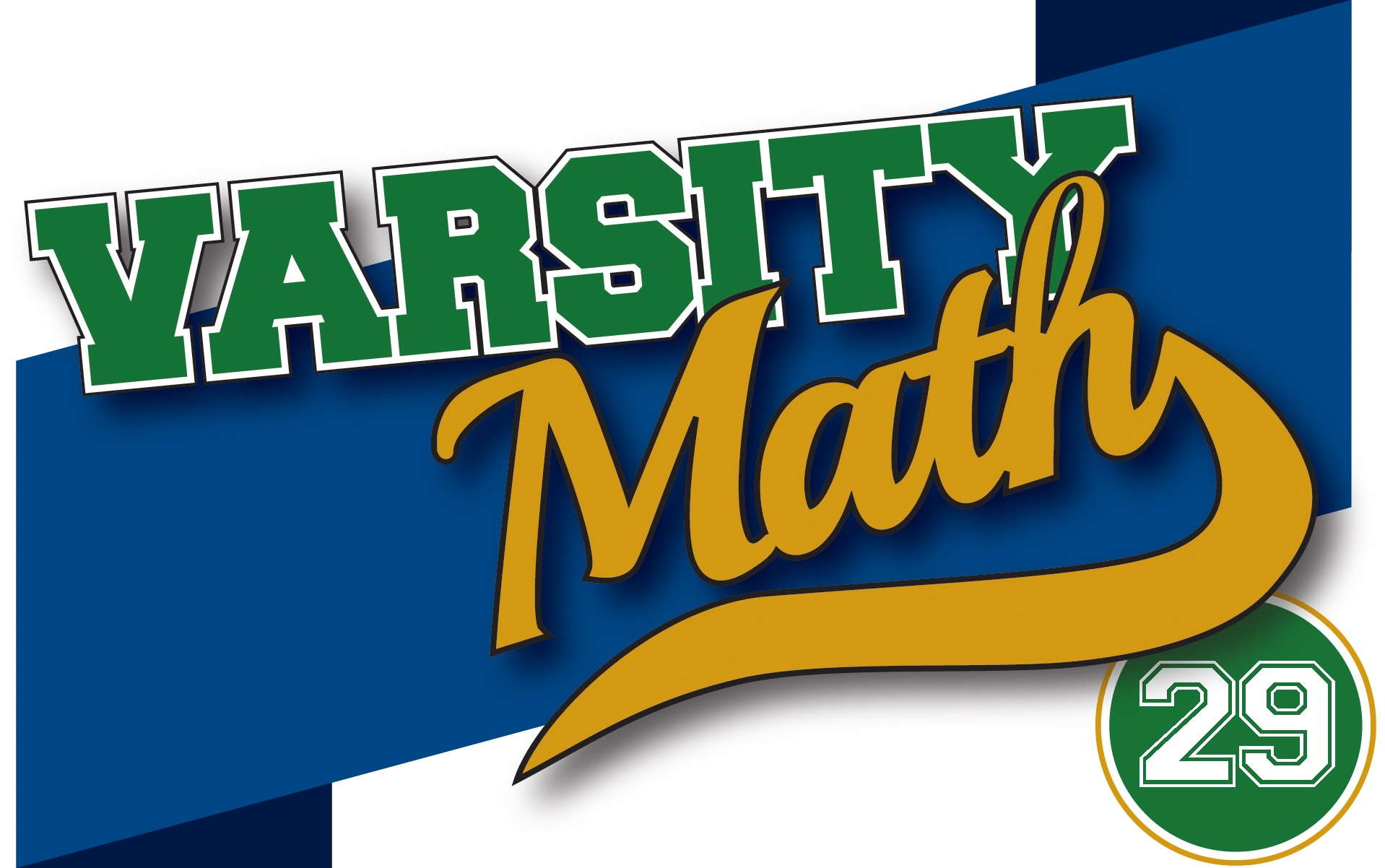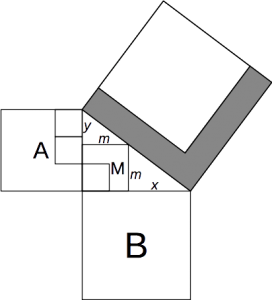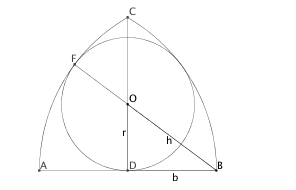Geometry — both abstract and practical — is on the mind of the Varsity Math team this week.### Patriotic Packing

The Varsity Math team is helping pack up the red, white, and blue glassware from a Fourth of July celebration. Each glass is a perfect cylinder, two inches in diameter. They have boxes that are a foot wide and 16 inches long, and the same height as the glasses.

What is the maximum number of glasses that can be packed into one of the boxes, assuming all of the glasses are standing upright?

### Talking Midpoints

You are given a set S of 24 distinct points in the plane with integer coordinates. Form the set M of all of the midpoints of line segments that have both endpoints in S. Assume that no two such segments have the same midpoint, i.e., that M has the maximum possible number of members.

What is the minimum possible number of members of M that have only integer coordinates?

## Solutions to Week 28

Inscribed Square.Given that the area of square B is 16, the large central triangle is a 3-4-5 triangle, and we want to find the area, and hence the side length m, of the inscribed square M. But if we let x and y be the portions of the legs “left over” from M as shown, then we have that m = 4 – x and that m = 3 – y. Also, the two smaller right triangles above and to the right of M are similar, so y / m = m / x. Substituting and solving, we have that (3 – m) / m = m / (4 – m), so m² = 12 – 7m + m² and m = 12/7, so the the desired area of M is 144/49.

Gothic Arc.The diagram shows the configuration as described in the problem. Note that since the arc AC is tangent to the circle U at the point F, the arc and circle have the same tangent line and hence the same perpendicular to the tangent. Hence the line through the center O of circle U coincides with the line through the center B of arc AC as shown. Similarly, the perpendicular bisector of segment AB also goes through O. So let the radius of circle U be r, the other leg of right triangle ODB be b, and the hypotenuse of that triangle be h. Since arc radius AB equals radius FB and OF is another radius of circle U and hence of length r, we have that r + h = 2 b. Also, by the Pythagorean Theorem (rearranged slightly), we have r² – h² = –b². Dividing the latter equation by the former, we get that r – h = –b/2. Adding to the first equation, we get that 2r = 3b/2. Since b is half the radius of arc AC, we get that the radius of circle U is 3/8 of the radius of arc AC. And since arc AC is one-sixth of a full circle, the circumference of circle U is 6 × 3/8 = 9/4 of the length of arc AC. Looking back at the answer to Inscribed Square, we see that the length of arc AC is 12, so the circumference of circle U is 12 × 9/4 = 27.

Midpoint Well Taken. Let p and q be the points in S (which has 27 elements) which are farthest apart. The 26 midpoints of p and another point in S are all different. The 26 midpoints of q and another point in S are all different. Moreover, the only overlap between these two groups of midpoints is the midpoint of pq. To see why, suppose that midpoint px coincided with midpoint qy. Then pqxy is a parallelogram, and one of the diagonals of any parallelogram is longer than any side, so either px or qy is longer than pq, contradicting that p and q were the farthest apart. Thus, we have shown there are at least 51 different midpoints. On the other hand, if the points of S are just the points (0,0), (1,0), …, (26,0), then the midpoints are just (1/2, 0), (2/2, 0), …, (51/2, 0). In other words, the minimum possible number of midpoints is 51.

Closing Times. It does not have to be the case that both the greens and blues are closed; for example take the greens to be the negative numbers and the blues to be the positive numbers and zero. Then any product of three negatives is negative, and any product of three positive/zero numbers is either positive or zero, but the product of two negatives is not negative. On the other hand, whichever color 1 is must be closed under multiplication, because if x and y are any two points of that color, then xy = 1xy is also that color.

## Recent Weeks

Links to all of the puzzles and solutions are on the Complete Varsity Math page.

Come back next week for answers and more puzzles.